Courses

# Rigid Body Dynamics MSQ

## 10 Questions MCQ Test Basic Physics for IIT JAM | Rigid Body Dynamics MSQ

Description
This mock test of Rigid Body Dynamics MSQ for Physics helps you for every Physics entrance exam. This contains 10 Multiple Choice Questions for Physics Rigid Body Dynamics MSQ (mcq) to study with solutions a complete question bank. The solved questions answers in this Rigid Body Dynamics MSQ quiz give you a good mix of easy questions and tough questions. Physics students definitely take this Rigid Body Dynamics MSQ exercise for a better result in the exam. You can find other Rigid Body Dynamics MSQ extra questions, long questions & short questions for Physics on EduRev as well by searching above.
*Multiple options can be correct
QUESTION: 1

### In the shown figure, the pulley of mass M and radius R can rotate about its fixed horizontal axis (axle) without friction. Friction between light inextensible string and pulley is sufficient to prevent slipping of string over pulley. The masses of blocks are m1 and m2 such that m2 > m1. The system is released from rest as shown. Before the block of mass m1 touches the pulley, pick up the correct statements.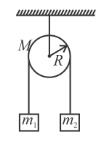Solution:

The acceleration of three sections of rope 1, 2, 3 are as shown.

Hence for the section of rope in contact with pulley, acceleration increases till the section looses contact with pulley.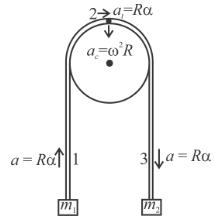Due to friction between string and pulley, the tension in right portion of string is larger in comparison to tension in the left portion of string.

The correct answers are: Magnitude of force exerted by string on mass m2 is larger as compared to that exerted by string on mass  m1., The acceleration of small length dℓ of string in contact with block of mass m2 remains constant.

*Multiple options can be correct
QUESTION: 2

### A smooth tube of certain mass is rotated in gravity free space and released. The two balls shown in the figure move towards ends of the tube. For the whole system which of the following quantity is conserved?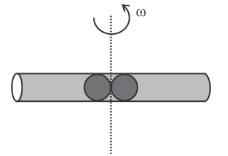Solution:

As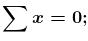Angular momentum, conserved. F = 0, linear momentum will remain compound.

As the two balls will move radially out, I changes. In order to keep the angular momentum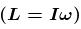conserved, angular speed (ω) should change.

The correct answers are: Angular momentum, Linear momentum, Kinetic energy

*Multiple options can be correct
QUESTION: 3

### A ladder AB is supported by a smooth vertical wall and rough horizontal floor as shown. A boy starts moving from A to B slowly. The ladder remains at rest, then pick up the correct statement(s).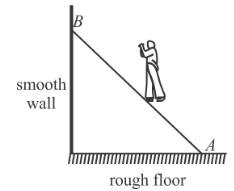Solution:

Let m and M be mass of man and ladder. From FBD normal reaction at A is N1 = (m + M)g which remains constant. Net torque on man + ladder is zero about B. If x decreases then torque of m about B will decrease.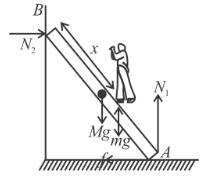Hence f must increases.

The correct answers are: Magnitude of normal reaction by wall on ladder at point B will increase., Magnitude of normal reaction by floor on ladder at point A will remain unchanged, Magnitude of friction force by floor on ladder at point A will increase

*Multiple options can be correct
QUESTION: 4

A uniform disc is performing pure rolling on a smooth stationary surface with constant angular velocity as shown in figure. At any instant, for the lower most point of the disc :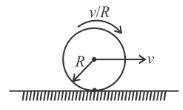Solution:

As the disc is in combined rotation and translation, each point has a tangential velocity and a linear velocity in the forward direction.

From figure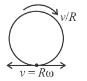vnet (for lower point) =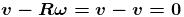and Acceleration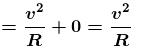(Since linear speed is constant

The correct answers are: Velocity is zero, acceleration is v2/R

*Multiple options can be correct
QUESTION: 5

A uniform rod AC of length ℓ and mass m is kept on a horizontal smooth plane. It is free to rotate and move. A particle of same mass m moving on the plane with velocity v strikes the rod at point B making and angle 37° with the rod. The collision is elastic. After collision :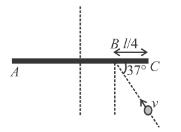Solution:

The ball has v', component of its velocity perpendicular to the length of rod immediately after the collision. u is velocity of COM of the rod and is angular velocity of the rod, just after collision. The ball strikes the rod with speed v cos 53° in perpendicular direction and its component along the length of the rod after the collision is unchanged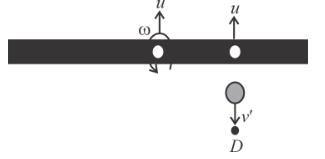using for the point of collision.

Velocity of separation = Velocity of approach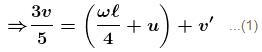Conserving linear momentum (of rod + particle), in the direction ⊥ to the rod.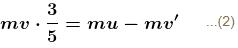Conserving angular moment about point ‘D’ as shown in the figure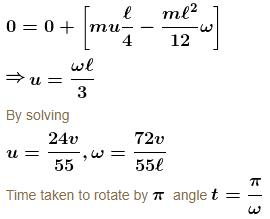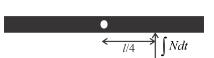In the same time, distance travelled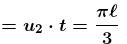Using angular impulse-angular momentum equation.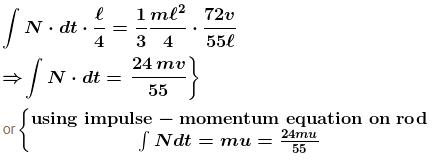The correct answers are: The angular velocity of the rod will be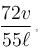The centre of the rod will travel a distance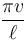in the time in which it makes half rotation, Impulse of the impact force is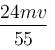*Multiple options can be correct
QUESTION: 6

A rigid body is in pure rotation, that is, undergoing fixed axis rotation. Then which of the following statement(s) are true?

Solution:

All points in the body in plane perpendicular to the axis of rotation revolve in concentric circles. All points lying on circle of same radius have same speed (and also same magnitude of acceleration) but different directions of velocity (also different directions of acceleration)

Hence there cannot be two points in the given plane with same velocity or with same acceleration.

As mentioned above, points lying on circle of same radius have same speed.

Angular speed of body at any instant w.r.t. any point on body is same by definition.

The correct answers are: Speed of all the particles lying on the curved surface of a cylinder whose axis coincides with the axis of rotation is same., Angular speed of the body is same as seen from any point in the body

*Multiple options can be correct
QUESTION: 7

A uniform disc of mass 2kg and radius 1m is mounted on an axle supported on fixed frictionless bearings. A light cord is wrapped around the rim of the disc and mass of 1kg is tied to the free end. If it is released from rest :

Solution:

By FBD of particular
mg – T = ma
10 – T = a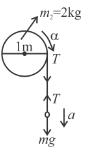BY FBD of disc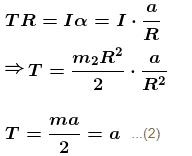By equation (1) and (2)
a = 5 m/s2
and T = 5 N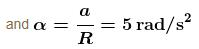For angular displacement of disc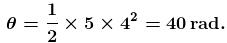Work done by torque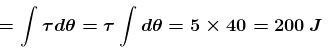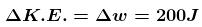K2 – K1 = 200J

The correct answers are: the tension in the cord is 5 N, in first 4 seconds the angular displacement of the disc is 40 rad., the work done by the torque on the disc in first 4 sec. is 200 J, the increase in the kinetic energy of the disc in the first 4 seconds is 200 J

*Multiple options can be correct
QUESTION: 8

A uniform bar of length 6a and mass 8m lies on a smooth horizontal table. Two point masses m and 2m moving in the same horizontal plane with speeds 2v and v respectively strike the bar as shown in the figure and stick to the bar after collision.
Velocity of the centre of mass of the system is :

Solution:

(i) Conserving linear momentum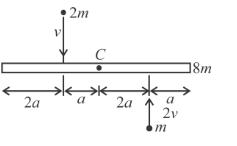–2mv + 2v.m = 0 = MBcm
Vcm = 0
(ii) As ball stick to Rod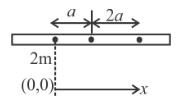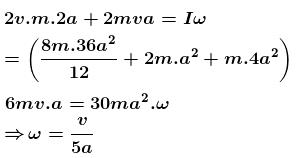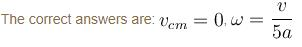*Multiple options can be correct
QUESTION: 9

A ball of mass m moving with constant velocity u collides with a smooth horizontal surface at O as shown. Neglect gravity and friction. The y-axis is drawn normal to the horizontal surface at the point of impact O and x-axis is horizontal as shown in the figure. About which point will the angular momentum of ball is not conserved.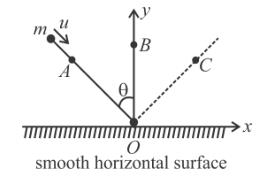Solution:

As the normal force exerted by horizontal surface passes through point B, external torque on the ball is zero about point B. So angular momentum of ball is conserved about point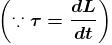The correct answers are: point A, point C

*Multiple options can be correct
QUESTION: 10

In the pulley system shown, if radii of the bigger and smaller pulley are 2m and 1m respectively and the acceleration of block A is 5 m/s2 in the downward direction, then :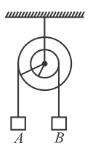Solution: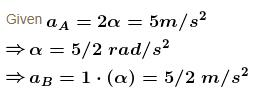The correct answers are: Angular acceleration is 2.5 rad/s2, Acceleration of B is 2.5 m/s2